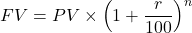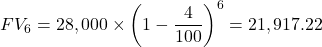## Colin buys a car for £28100. It depreciates at a rate of 4% per year. How much will it be worth in 6 years? Give your a

Question

Colin buys a car for £28100.
It depreciates at a rate of 4% per year.
How much will it be worth in 6 years?

in progress 0
5 months 2021-08-23T10:52:36+00:00 1 Answers 5 views 0

The amount the car will be worth after 6 years is £21,917 and 22 pence

Step-by-step explanation:

The amount for which Colin buys the car, PV = £28,100

The amount by which the car depreciates each year, r = 4%

The number of years after which the value of the car is sought, n = 6 years

The future value, FV, based on an annual depreciation is given as follows;Substituting the known values gives;Therefore, the amount the car will be worth after 6 years, future value FV₆ = £21,917 and 22 pence